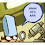## Wednesday, June 24, 2009

### Two requets: A sum and a reference

I have two requests from my readers. Both are essentially help tracking things down.

First: We (Bill Gasarch, Clyde Kruskal, Justin Kruskal) have in a paper of ours a summation that we proved. Is it new? Here is the summation: (It uses a sum that I previously inquired about here and got useful information, including that it was originally proved by Euler.)

Let p(x) &isin Z[x] be a polynomial of degree n with constant term 0.

p(s) - &sumk=1n(s+k-1 choose k)&sumi=0k-1(k-1 choose i)(-1)i (p(s+i+1) - p(s+i)) = 0
Our proof is here.

Second: I am, as always, tracking down lower bounds on the VDW numbers. I need the article An application of the Lovasz Local Lemma, a new lower bound for the Van der Warden numbers by Zoltan Szabo, Random Structures and Algorithms, 1990. Electronic preferred, but will take what you got. SO, if someone has it please email me article or pointer or whatnot. (Pointer might not work if your library has access to this journal, since ours does not have it in electronic or hardcopy.)

1.You don't. Instead you install a LaTeX script on your blog, e.g. LaTeXMathML.

2.I Recommend itex2mml for translation. It'll change itex (a subset of LaTeX) into MathML. See:
itex2mml

3.<?xml version="1.0" encoding="UTF-8"?>
<!DOCTYPE math:math PUBLIC "-//OpenOffice.org//DTD Modified W3C MathML 1.01//EN" "math.dtd">
<math:math xmlns:math="http://www.w3.org/1998/Math/MathML">
<math:semantics>
<math:mfenced math:open="" math:close="">
<math:mtable>
<math:mtr>
<math:mrow>
<math:mi>x</math:mi>
<math:mo math:stretchy="false">−</math:mo>
<math:mn>1</math:mn>
</math:mrow>
</math:mtr>
<math:mtr>
<math:mi>x</math:mi>
</math:mtr>
</math:mtable>
</math:mfenced>
</math:semantics>
</math:math>

4.This identity is not new. There is a procedure called the method of difference due to pascal in order to compute/approximate functions. It is known to work exactly for polynomials and that boils down to this identity.

5.the e-version of the article is available from UCB library. My UC campus cannot access it. but if you dont get it please leave a note here and I will try through the library.

6.sep322,

Yup, that's why you use a conversion script rather than writing MathML by hand.

Anonymous,

itex2mml is great, but for a blog where you just want to write short snippets of math it is easier to go with a javascript solution that you can just add into the HTML header of your blog. That's why I would recommend LaTeXMathML. It is not perfect, but until a better solution comes along it is the best that I know of.

Of course, if you are using Wordpress or Moveable Type then you can use Jacques Distler's plugin based on itex2Mmml, but there isn't one for Blogger as far as I know.

Another thing worth mentioning is that there are a few graphical MathML editors available (a bit like Microsoft Equation Editor) that produce MathML directly. They may be suitable if you only need to do this sort of thing occasionally.

Finally, it is also the case that you can find several plugins for converting LaTeX equations into .png images if you feel like you absolutely have to (but don't because you don't).

7.Anon who offered to try to get me the article I want- Nobody has
gotten me the article yet, so yes

Thanks

BIll Gasarch
(logged in to a dif person so it
will look like its Anon but its not)

8.No idea about prior art, but that looks an awful lot like the discrete calculus analog of the fact that the $n$th degree Taylor expansion of a polynomial of degree $n$ is the polynomial.

9.One of my readers emailed me the
paper. Actually it was Lance
(I think he reads my blogs).

Thanks Lance and thanks Anon who
offered to help.

10.I think this is the Newton series for the difference operator.

11.Bill,
FYI, the advantage of an article NOT appearing in hardcopy on the UM system is that they'll get it interlibrary loan for you... and scan it in and email it to you. I've started hoping that they never have the articles I want in hardcopy...

12.The first lemma in your proof is a direct consequence of the method of finite differences; it says that the nth forward difference of a polynomial of degree n-1 is identically zero.

The inner summation in the second identity is basically computing the coefficients of the "Newton form" of the polynomial, although you seem to be using a slightly different basis. Nevertheless, these results are quite standard.